Processing ......FreeComputerBooks.com Links to Free Computer, Mathematics, Technical Books all over the World

Revealing Statistical Principles
Top Free Algorithms Books 🌠 - 100% Free or Open Source!
• Title: Revealing Statistical Principles
• Author(s) Jim K. Lindsey
• Publisher: Hodder Education Publishers
• Paperback: 232 pages
• eBook: PDF, ePub, Kindle, etc.
• Languages: English, Belorussian
• ISBN-10: 0340741201
• ISBN-13: 978-0340741207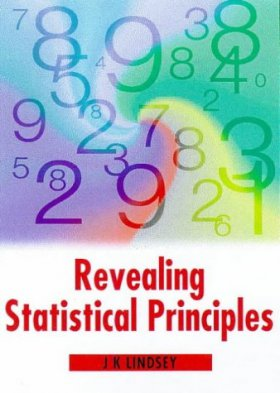Book Description

Written by a renowned statistician, this book presents the basic ideas behind the statistical methods commonly used in studies of human subjects. It is an ideal guide for advanced undergraduates who are beginning to do their own research. It presents the basic principles in a non-mathematical way and is accessible to a wide audience with little background in statistics. It collects a large amount of material scattered throughout the literature and will be an invaluable tool for projects in a wide range of disciplines.

Accessible to a wide audience with little background in statistics, the book outlines the necessary steps to conduct research on human subjects. Through a series of questions and checklists, it carries the reader through each stage of the research project. The book contains very detailed and practical considerations for research design which are likely to be overlooked by novice researchers (and perhaps even a few statisticians).

• Jim Lindsey, Department of Biostatistics, Limburgs University, Belgium.
Reviews, Ratings, and Recommendations: Related Book Categories: Read and Download Links:Similar Books:
•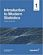Introduction to Modern Statistics (Mine Çetinkaya-Rundel, et al.)

This book puts a heavy emphasis on exploratory data analysis and provides a thorough discussion of simulation-based inference using randomization and bootstrapping, followed by a presentation of the related Central Limit Theorem based approaches.

•Foundations in Statistical Reasoning (Pete Kaslik)

This book is designed for students taking an introductory statistics class. The emphasis throughout the entire book is on how to make decisions with only partial evidence. It focuses on the thought process.

•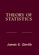Theory of Statistics (James E. Gentle)

This book is directed toward students for whom mathematical statistics is or will become an important part of their lives. It covers classical likelihood, Bayesian, and permutation inference; an introduction to basic asymptotic distribution theory; and modern topics.

•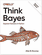O'Reilly® Think Bayes: Bayesian Statistics in Python

If you know how to program, you're ready to tackle Bayesian statistics. With this book, you'll learn how to solve statistical problems with Python code instead of mathematical formulas, using discrete probability distributions rather than continuous mathematics.

•Statistical Inference: Algorithms, Evidence, and Data Science

A masterful guide to how the inferential bases of classical statistics can provide a principled disciplinary frame for the data science of the twenty-first century. Every aspiring data scientist should carefully study this book, use it as a reference.

•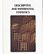Foundations of Descriptive and Inferential Statistics (H. van Elst)

This book aim to provide an accessible though technically solid introduction to the logic of systematical analyses of statistical data to both undergraduate and postgraduate students, in particular in the Social Sciences, Economics, and the Financial Services.

•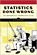Statistics Done Wrong: The Woefully Complete Guide (Reinhart)

Scientific progress depends on good research, and good research needs good statistics. But statistical analysis is tricky to get right, even for the best and brightest of us. You'd be surprised how many scientists are doing it wrong.

•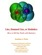Lies, Damned Lies: How to Tell the Truth with Statistics

The goal is to help you learn How to Tell the Truth with Statistics and, therefore, how to tell when others are telling the truth ... or are faking their "news". Covers Data Analysis, Binomial and normal models, Sample statistics, confidence intervals, hypothesis tests, etc.

•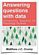Answering Questions with Data : Introductory Statistics

This is a free textbook teaching introductory statistics for undergraduates. Students will learn to select an appropriate data analysis technique, carry out the analysis, and draw appropriate conclusions.

•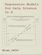Regression Models for Data Science in R (Brian Caffo)

The book gives a rigorous treatment of the elementary concepts of regression models from a practical perspective. The ideal reader for this book will be quantitatively literate and has a basic understanding of statistical concepts and R programming.

•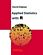Applied Statistics with R (David Dalpiaz)

This book provides an integrated treatment of statistical inference techniques in data science using the R Statistical Software. It provides a much-needed, easy-to-follow introduction to statistics and the R programming language.

•Statistical Methodologies and Their Application to Real Problems

This book provides a cross-disciplinary forum for exploring the variety of new data analysis techniques emerging from different fields, focusing on recent advances in data analysis techniques in many different research fields.

•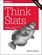Think Stats, 2nd Edition: Exploratory Data Analysis in Python

This concise introduction shows you how to perform statistical analysis computationally, rather than mathematically, with programs written in Python. You'll become familiar with distributions, the rules of probability, visualization, and many other tools and concepts.

•Introduction to the Theory of Statistics (Alexander M. Mood, et al)

This book is a self contained introduction to classical statistical theory. The material is suitable for students who have successfully completed a single year's course in calculus with no prior knowledge of statistics or probability.

•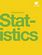Introductory Statistics (OpenStax College)

This book is geared toward students majoring in fields other than math or engineering. This text assumes students have been exposed to intermediate algebra, and it focuses on the applications of statistical knowledge rather than the theory behind it.

•Seeing Theory: A Visual Introduction to Probability and Statistics

The goal of this book is to make statistics more accessible through interactive visualizations (using D3.js). It visualizes the fundamental concepts covered in an introductory college statistics or Advanced Placement statistics class.

•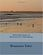Introduction to Statistical Thinking, with R, without Calculus

This is an introduction to statistics, with R, without calculus, for students who are required to learn statistics, students with little background in mathematics and often no motivation to learn more.

Book Categories
 :All CategoriesTop Free BooksRecent BooksMiscellaneous BooksComputer EngineeringComputer LanguagesComputer ScienceData Science/DatabasesJava and Java EE (J2EE)Linux and UnixMathematicsMicrosoft and .NETMobile ComputingNetworking and CommunicationsSoftware EngineeringSpecial TopicsWeb Programming
Other Categories